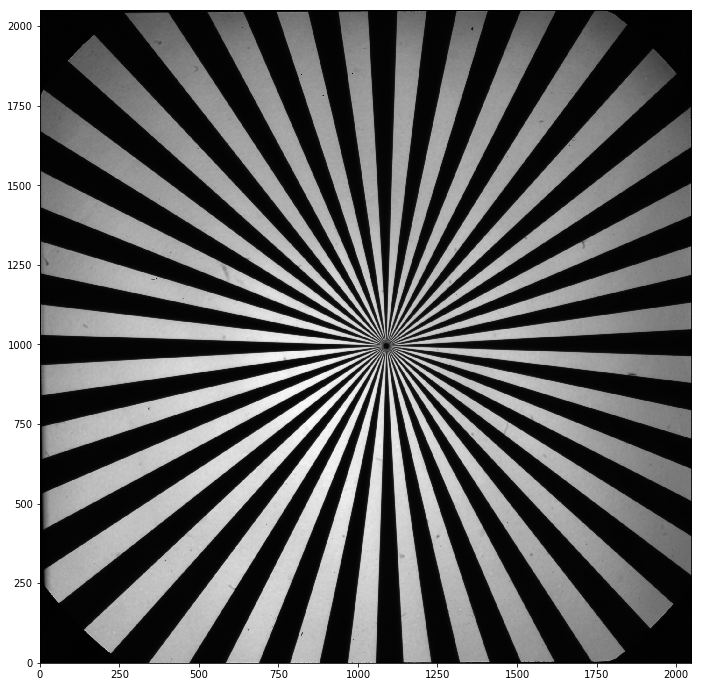# How to use the Siemens Star calibration Target to obtain the MTF of an optical system

## Siemens Star Analysis¶

### In this notebook I wil show how to use the Siemens Star Target to characterize the Modulation Transfer Function of an optical system.¶

In :
```import numpy as np
import pyfits
import matplotlib.pyplot as plt
import skimage
from skimage.feature import blob_dog, blob_doh, blob_log, canny
from skimage.color import rgb2gray
from skimage.feature import corner_harris, corner_subpix, corner_peaks
from skimage.segmentation import slic
from skimage.filters import sobel
from scipy.signal import convolve2d

from scipy.ndimage import gaussian_filter
from skimage import measure
from scipy.optimize import curve_fit
import matplotlib.ticker as mtick
from scipy.signal import savgol_filter
```
In :
```path = '/home/fatima/Desktop/solar_orbiter_project/codes/targets/MTF/'
```
In :
```star = pyfits.getdata(path+'solo_L0_1805230011_20180523T121104.fits')
dark = pyfits.getdata(path+'solo_L0_1805230009_20180523T094103.fits')
data = star/20. -dark/20.
```
In :
```fig = plt.figure(figsize=(18,12))

plt.imshow(data,origin='lower',cmap='gray')
```
Out:
`<matplotlib.image.AxesImage at 0x7f4556699950>`## How to compute the contrast modulation?¶

#### The idea is simple, we draw concentric circles around the origin and build the profile along its edges. From this profile (of a frequrency that we know), we compute the contrast modulation. The frequency will be radius dependent and is simply the ratio of the number of line pairs (number of white-black stripes) over the length of the circle. Let's define some functions.¶

In :
```## this function will get the values along each circle. We start by defining a range of angles, computing the
#coordinates and then finding the values at the nearest pixel position.

#theta = np.linspace(0,2*np.pi,N_theta)
x = np.round(x)
y = np.round(y)
x = x.astype(int)
y = y.astype(int)
I = star[y,x]

return I,x,y
```
In :
```## a function to compute the frequecy for a certain radius
N_p = 36
r = N_p/(2*np.pi*freq)
return r
## a function to compute the radius for a certain frequency
N_p = 36
return freq
```

#### Now let's draw these circles around the center¶

In :
```shape = data.shape
R_max = 1000
N_theta = 300
theta = np.linspace(0,2*np.pi,N_theta)
N_p = 36      #angular frequency (number of dark (bright) lines)
# center of the target (determined manually)
x_c = 1089
y_c =996
```
In :
```plt.figure(figsize=(18,12))
plt.imshow(data, origin='lower')